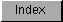```
Utility library contains some high-speed math functions for 32-bit integer
division and multiplication. These functions will take advantage of
available processor instructions (like DIVUL), if a 68020 processor or
higher is present. If not, these functions will mimic those instructions
in 68000 only instructions, thus providing processor independency.

Currently the following functions are implemented:

____________________________________________________________
|                                                            |
|  SDivMod32()  Signed 32 by 32-bit division and modulus.    |
|    SMult32()  Signed 32 by 32-bit multiplication.          |
|  UDivMod32()  Unsigned 32 by 32-bit division and modulus.  |
|    UMult32()  Unsigned 32 by 32-bit multiplication.        |
|____________________________________________________________|

Table 37-3: Utility Library 32-bit Math Functions

The division functions return the quotient in D0 and the remainder in D1.
To obtain the remainder in a higher level language, either a compiler
specific instruction to fetch the contents of a specific register must be
used (like getreg() in SAS C) or a small assembler stub.

Following a simple example of the usage of the 32-bit integer math
functions in C.

uptime.c

```